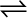7.27 The equilibrium constant for the following reaction is 1.6 ×105 at 1024K

H2(g) + Br2(g)2HBr(g)

Find the equilibrium pressure of all gases if 10.0 bar of HBr is introduced into a sealed container at 1024K.

NEET SOLUTION:

Given,
${\mathrm{K}}_{\mathrm{p}}$ for the reaction i.e.,
Therefore, for the reaction, the equilibrium constant will be,
$\mathrm{K}{\text{'}}_{\mathrm{p}}=\frac{1}{{\mathrm{K}}_{\mathrm{p}}}\phantom{\rule{0ex}{0ex}}=\frac{1}{1.6×{10}^{5}}\phantom{\rule{0ex}{0ex}}=6.25×{10}^{-6}$
Given,
Now, let p be the pressure of both  a equilibrium.
Now, we can write,
Therefore, at equilibrium,
$\left[{\mathrm{H}}_{2}\right]=\left[{\mathrm{Br}}_{2}\right]=2.49×{10}^{-2}\mathrm{bar}\phantom{\rule{0ex}{0ex}}\left[\mathrm{HBr}\right]=10-2×\left(2.49×{10}^{-2}\right)\mathrm{bar}\phantom{\rule{0ex}{0ex}}=9.95\mathrm{bar}=10\mathrm{bar}\left(\mathrm{approx}.\right)$